## Introduction

An algebraic expression is a mathematical statement containing variables. Examples: 2x, 7p2 – 3p + 2, 4m3 + 6n – 1, etc.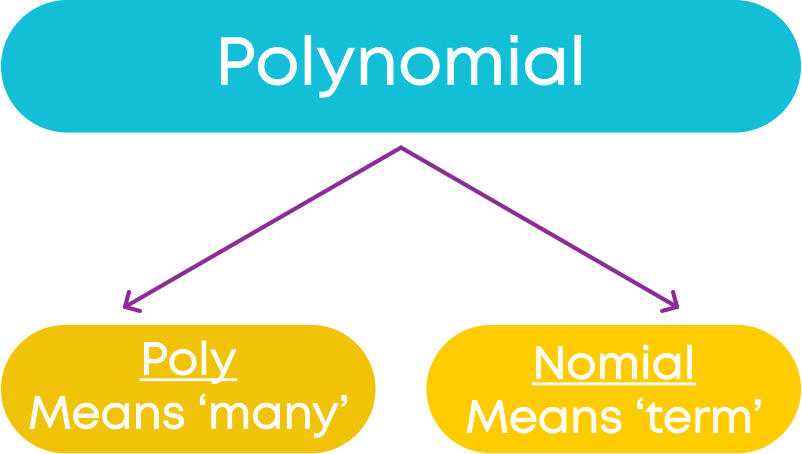As the name says, a polynomial contains many terms. But we can have a polynomial with one term also. It can be a constant as well. A polynomial can have any number of terms. Examples of polynomials: 3y – 2, 6a2 + 5a + 3, 7x4 + 11, 9t, 17

To explore more watch the video given below:

Rollercoasters are built by project/structural engineers using complex calculations. To model all the curves and ups and downs of the rollercoaster, they use polynomials. Civil engineers also use polynomials to design roads, buildings, and other structures.

Even medical researchers have found polynomials useful in describing the behaviour of bacterial colonies.

#### Concepts

The chapter ‘Polynomials’ covers the following concepts:

#### Polynomials in One Variable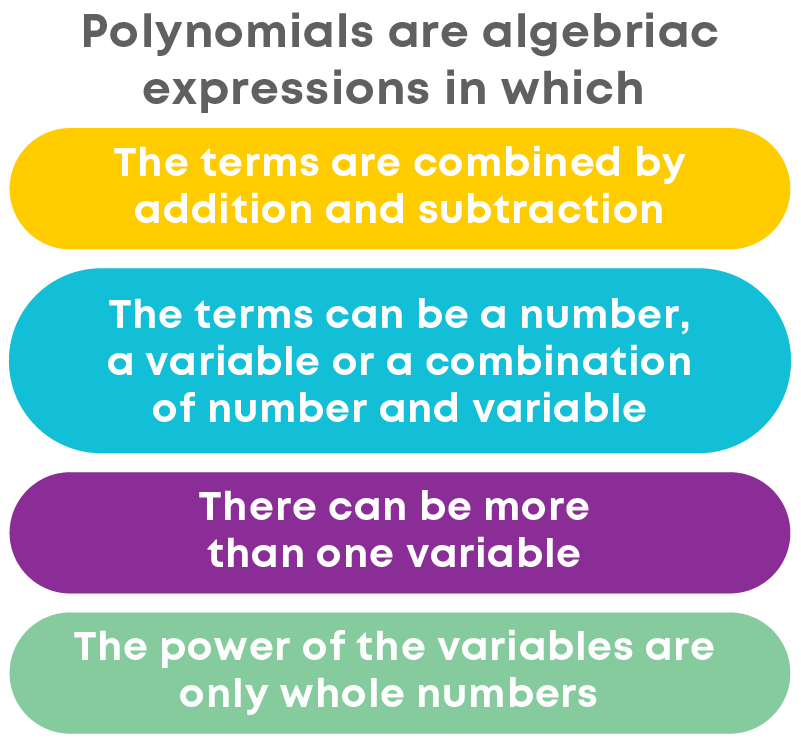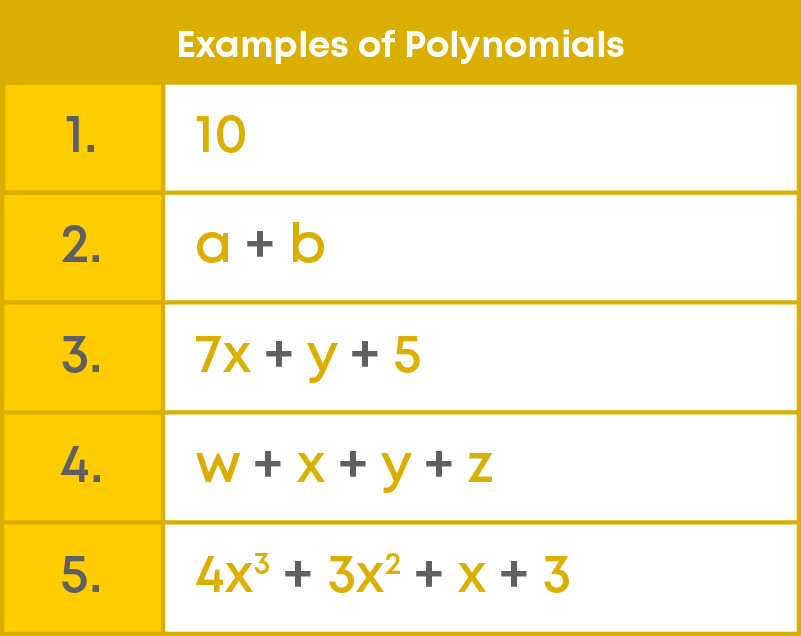Consider the following expression:

2√x – 3y + 17

Observe the variables in the expression. What is the power of ‘x’? In the expression, we can see that the power of the variable ‘x’ is 1 2 which is not a whole number. Can we say this is a polynomial? No. It is an algebraic expression but not a polynomial.

Let us understand the difference between an algebraic expression and a polynomial.

An algebraic expression can be described as a combination of constants and variables with four mathematical operations: addition, subtraction, multiplication, and/or division.

An algebraic expression can take any real value for exponents, so √x is an algebraic expression but not a polynomial. Also, the powers of the variables of an algebraic expression can be fractional/rational or negative.

Polynomial is an expression in which the variables have only positive integral powers. Examples: 5x, -5x + 3, 4x2 + 9x + 7

Some more examples are given below: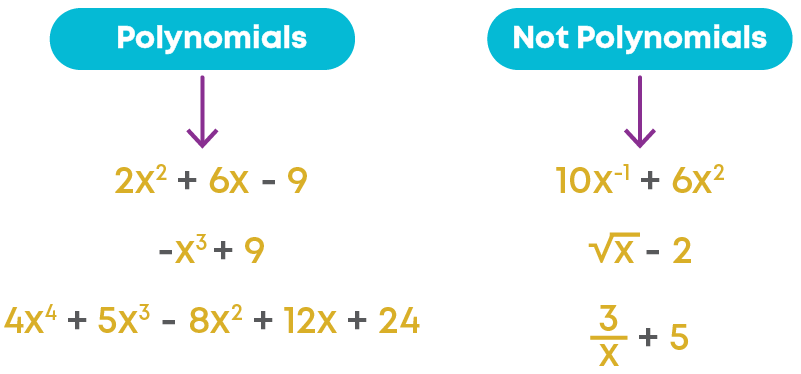Suppose you and your friend play a game. In the game you both put coins in a jar. Your friend puts thrice the number of coins put by you. Note: Every time the same number of coins are put in the jar.After 5 rounds, you want to find the number of coins in the jar. Suppose that you put ‘x’ number of coins and your friend puts 3x number of coins each time.

After the first round: x + 3x

After the second round: 2(x + 3x)

Similarly, after 5 rounds, we have the following equation.

5(x + 3x) = 5x + 15x = 20x

Here, given the value of ‘x’, we can find the number of coins. This is an example of polynomial in one variable, where the variable is ‘x’.

Polynomials in one variable are algebraic expressions that consist of terms in the form axn where n is a non-negative (i.e., positive or zero) integer and a is a real number.

If the variable in a polynomial is x, we may denote the polynomial by p(x) or q(x) or r(x), etc. The general form of a polynomial in one variable (say ‘x’) is given as: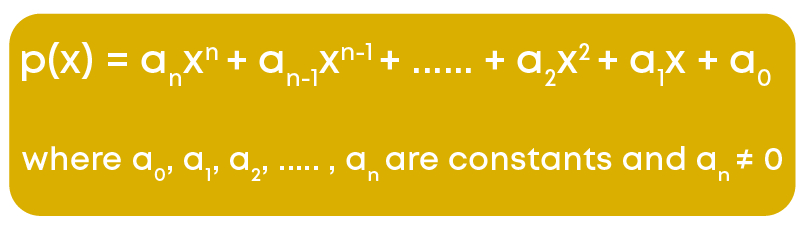For example: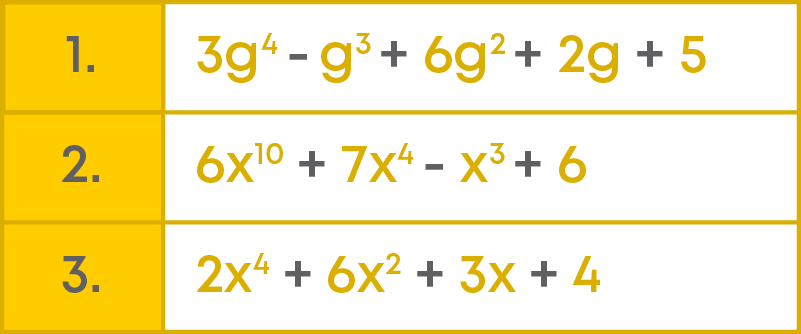Let us take a polynomial with variable ‘x.’

x3 – 2x + 3

We can write it as p(x) = x3 – 2x + 3

Consider the polynomials,

1) x2 + 2x

x2 and 2x are called the terms of the polynomial.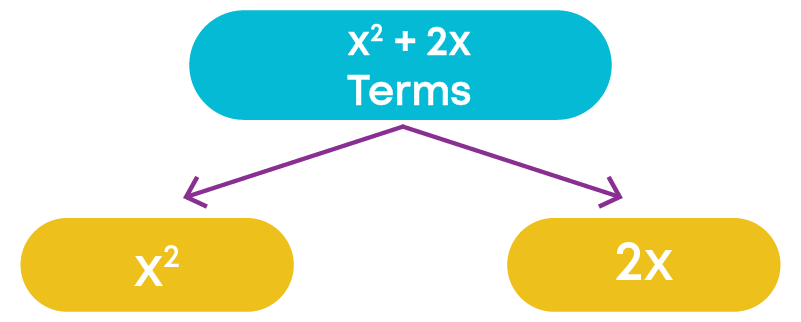2) 3y2 + 5y + 7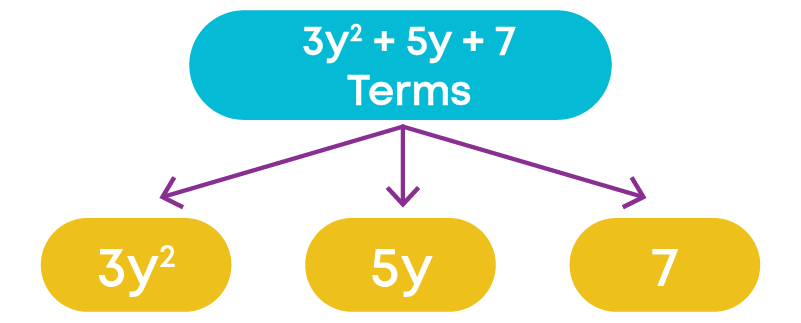Similarly, the polynomial 3y2 + 5y + 7 has three terms, namely 3y2, 5y, and 7.

Each term of a polynomial has a coefficient.

In x2 + 2x,

• The coefficient of x2 is 1
• The coefficient of x is 2

In 3y2 + 5y + 7

• The coefficient of y2 is 3
• The coefficient of y is 5
• 7 is the coefficient of y0 (remember, y0 = 1)

7 is called a constant term of the polynomial.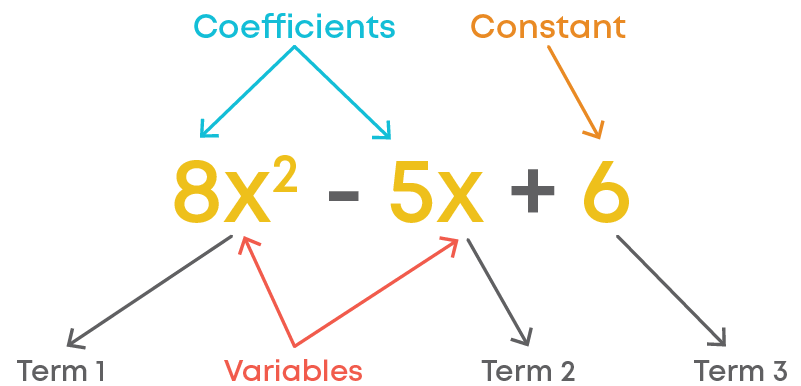A term is either a single number or a variable, and it can also be a combination of numbers and variables.

A combination of terms makes an expression. Terms are combined by addition operation. For example: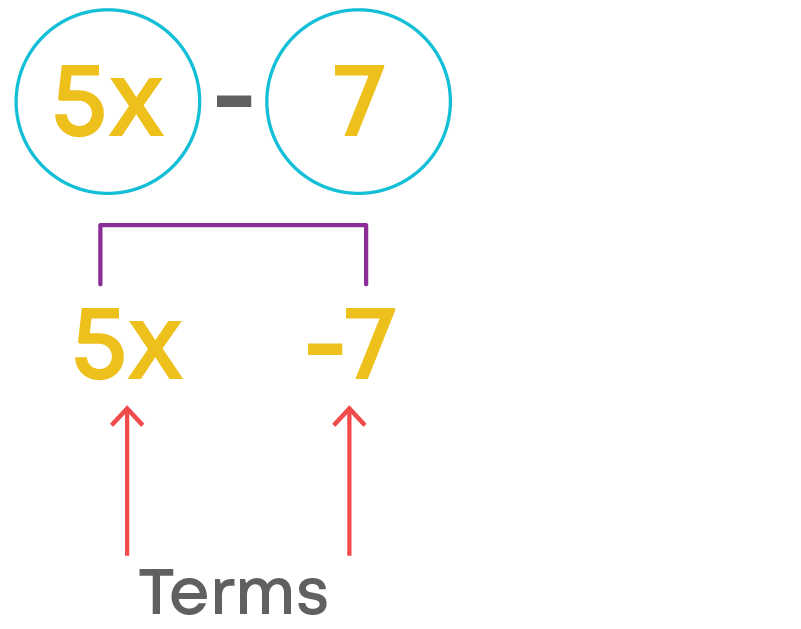The number that is multiplied with the variable (along with its sign, i.e., - or +) is called as the coefficient of the term.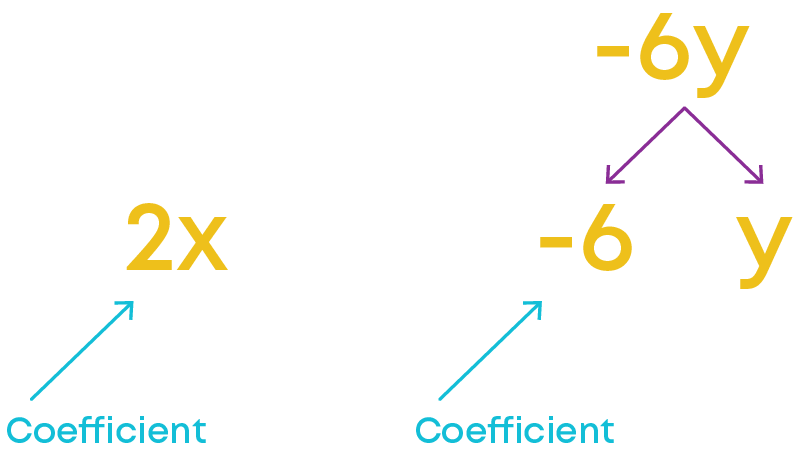Polynomials are classified based on:

• The number of terms
• The number of variables
• The degree of the polynomial

A polynomial can have any number of variables. Example, 7y + 3x3 – 8y2 + 2, x + y + z + r5

Depending on the number of variables, we can classify them as polynomials in one variable, polynomials in two variables, etc.

Based on the number of terms, polynomials are given special names.Monomials are polynomials with one term, hence the name monomial. For example, 4t, 21x2y, 9pq, are monomials because each of these polynomials contains only one term.

Binomials are polynomials with two unlike terms, hence the name binomial. For example, 3x + 4x2 is a binomial since it contains two unlike terms, that is, 3x and 4x2

Trinomials are polynomials with three unlike terms, hence the name Trinomial. For example: 3x + 5x2 – 6x3 is a trinomial because it contains three unlike terms, namely, 3x, 5x2, and -6x3.

Note: Polynomials can also be classified based on the highest power of the variable.

Now, let us have a look at some polynomials,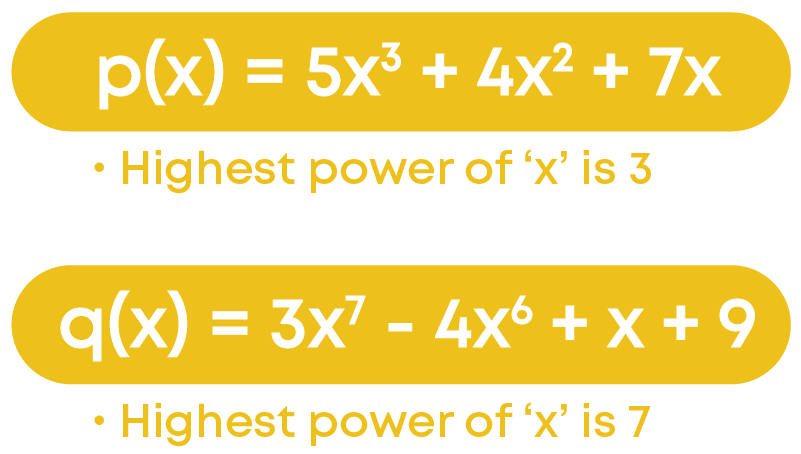The highest power of the variable in a polynomial is the degree of the polynomial. Therefore, the degree of polynomial,

• 5x3 + 4x2 + 7x is 3
• 3x7 - 4x6 + x + 9 is 7

Observe the given polynomials,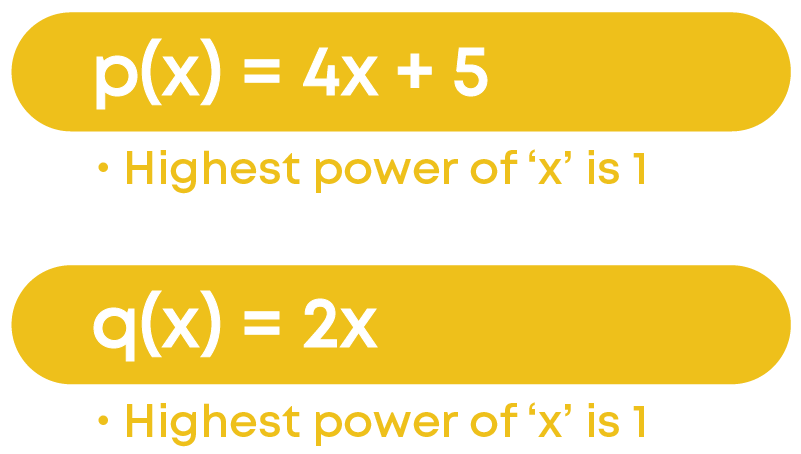The degree of each of these polynomials is one. A polynomial of degree one is called a linear polynomial.

Observe the given polynomials,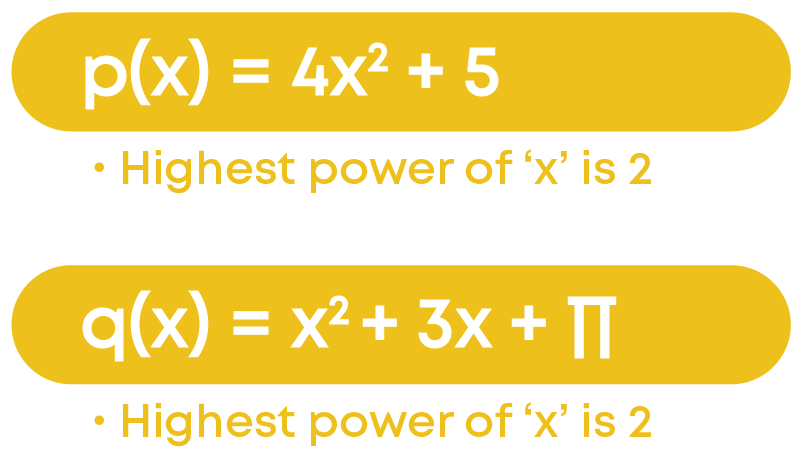Do you notice that these polynomials are all of degree two? A polynomial of degree two is called a quadratic polynomial.

Observe the given polynomials,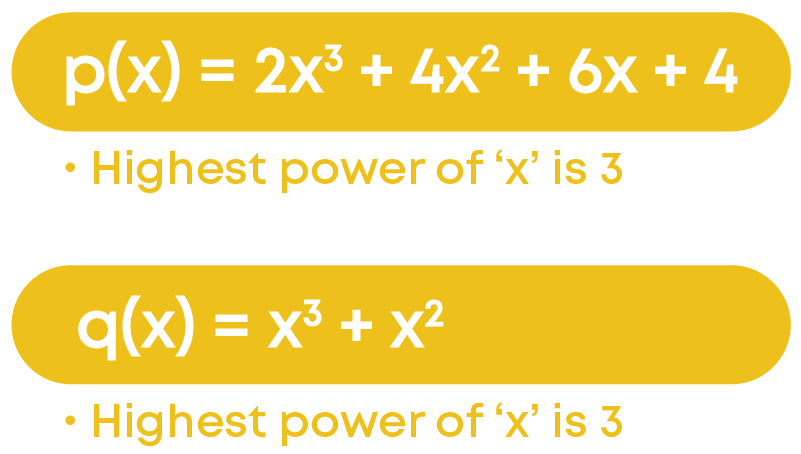Do you notice that these polynomials are all of degree three? We call a polynomial of degree three a cubic polynomial.

So, based on the degree, polynomials can be classified: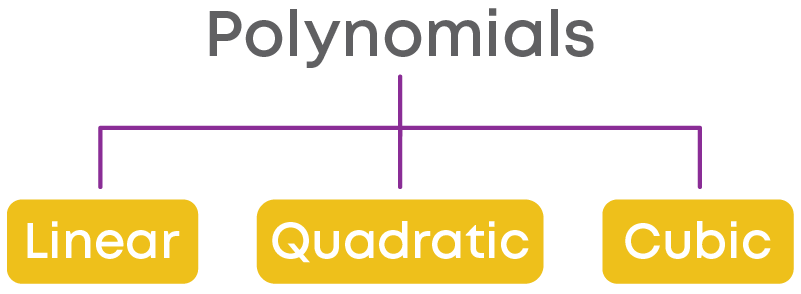The steps to find the degree of a polynomial are as follows:

For example, consider the polynomial:

4x5 + 7x3 + 3x2 + 5 + 8x

Step 1: Ignoring all the coefficients, the terms are x5, x3, x2, x0, x1

Step 2: Arrange them in the descending order of their powers.

x5, x3, x2, x0, x1

Step 3: The highest power of the variable is the degree of the polynomial.

Deg(4x5 + 7x3 + 3x2 + 5 + 8x) = 5

A polynomial consisting of only one constant is called a constant polynomial. Example, -7, 4, 2 3 , etc. are constant polynomials. Here, -7 can be written as -7x0.

Generally, each real number is a constant polynomial.

A constant polynomial is that whose value remains the same. It contains no variables. For example: p(x) = c.

We can see that there is no power here. Thus, the power of the constant polynomial is zero. Any constant can be written with a variable with the exponential power of zero. For example, the constant term 3 can be written in the polynomial form as p(x) = 3x0.

So, degree of a constant polynomial is 0. Is ‘0’ also a polynomial? Consider ‘0’. We can write ‘0’ as 0x0. We can see that ‘0’ can be expressed as a polynomial. A polynomial in which all the co-efficients are zero is called a zero polynomial. Now, what is the degree of the zero polynomial?

p(x) = 0

We can write it as,

• 0x0 – here the degree is 0

Or we can write it as,

• 0x3 – here the degree is 3

We can also write it as,

• 0x12 – here the degree is 12

What do you notice here? We can see that the degree of the zero polynomials is not fixed. Therefore, the degree of such polynomials is not defined.

So, a polynomial consisting of only one term, namely zero, is called a zero polynomial.

#### Zeroes of a Polynomial

Consider the polynomial p(x) = 4x3 – 2x2 + 3x + 3

If we replace ‘x’ by 2 in p(x), we get

p(2) = 4 × (2)3 – 2 × (2)2 + 3 × (2) + 3

= 32 – 8 + 6 + 3 = 33

We can say that the value of p(x) at x = 2 is 33.

Consider the given polynomial, p(x) = x – 5

If we substitute ‘x’ as 5, we get p(5) = 5 – 5 = 0. The value of the polynomial will be 0 at p = 5. So, 5 is called the zero of the polynomial.

Zeroes of the polynomial are the real values of the variable for which the value of the polynomial becomes zero.

From the previous examples, we see that a zero of a polynomial need not be always ‘0’ and ‘0’ may be a zero of a polynomial. Also, linear polynomials such as ax + b, 3x + 1, -7x and so on…has one and only one zero.

Let us verify whether 2 and ‘0’ are zeroes of the polynomial y2 – 2y.

Now we should follow the given steps:

• Step 1: Substitute the given value of variable in the given polynomial.
• Step 2: Simplify the expression.
• Step 3: Check if the value of the polynomial is zero.
• Step 4: If it is ‘0’, then the given value of the variable is a zero of the polynomial.

First, let us verify if 2 is a zero of the polynomial y2 – 2y.

Substitute y as 2:

p(y) = y2 – 2y

then, p(2) = (2)2 – 2(2) = 4 – 4 = 0

Now, let us verify if ‘0’ is the zero of the polynomial. Substitute y as 0:

p(0) = (0)2 – 2(0) = 0 – 0 = 0

Hence, 2 and 0 both are zeroes of the polynomial y2 – 2y.

Remember, a polynomial can have more than one zero.

#### Remainder Theorem

Let us recall factors and multiples: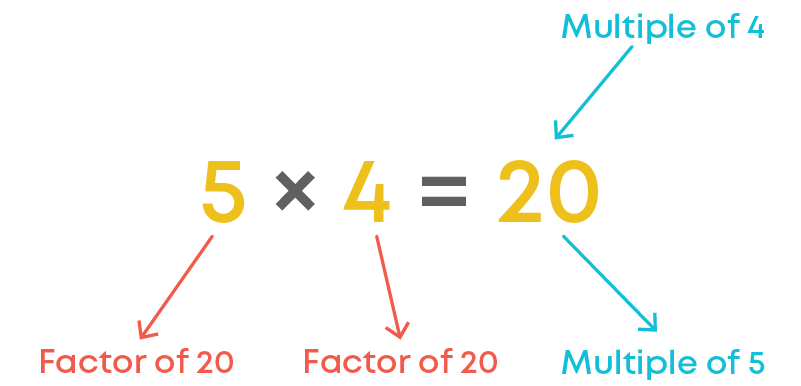A factor of the polynomial p(x) is any polynomial that divides p(x) completely, that is, the remainder is zero. For example, (x2 – 4) ÷ (x + 2) = x – 2 and the remainder is 0. So, x + 2 is a factor of the polynomial x2 – 4.

In general, given any polynomial p(x), a multiple of p(x) is any polynomial that can be factorised in the form p(x)q(x) for some polynomial q(x). Example: 4x3 + 3x2 + x = x × (4x2 + 3x + 1).

Here, 'x' and 4x2 + 3x + 1 are the factors of 4x3 + 3x2 + x.

Also, 4x3 + 3x2 + x is a multiple of ​'x' as well as a multiple of 4x2 + 3x + 1.

We follow the given steps to divide one polynomial by another polynomial:

• Step 1: We write the dividend and the divisor in the standard form, i.e., after arranging the terms in the descending order of their degrees. If any terms are missing, use a zero to fill in the missing term.
• Step 2: We divide the first term of the dividend by the first term of the divisor.
• Step 3: We multiply the divisor by the first term of the quotient and subtract this product from the dividend.
• Step 4: Bring down the next term and repeat the process till the remainder is ‘0’ or the degree of the new dividend is less than the degree of the divisor.

For example: x3-4x2+2x-3 x+2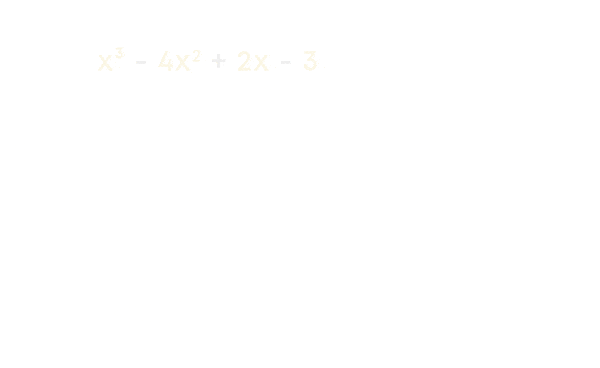The remainder theorem states that:

Let p(x) be any polynomial of degree greater than or equal to one and let ‘a’ be any real number. If p(x) is divided by the linear polynomial x – a, then the remainder is p(a).

Can we use the remainder theorem to find the remainder when a polynomial is divided by a linear polynomial? Yes!

By using the remainder theorem, the remainder after dividing p(x) by a linear polynomial g(x) can be found by finding the value of p(x) at the zero of g(x).

Consider the polynomial i.e., p(x) = x3 + x2 + 2x + 3 and linear polynomial g(x) = x + 2

g(x) = 0 → x + 2 = 0

x = -2

p(-2) = (-2)3 + (-2)2 + 2(-2) + 3 = -5

i.e., the zero of g(x) is -2 and the value of p(x) at -2 is -5.

So, according to the remainder theorem when the polynomial p(x) = x3 + x2 + 2x + 3 is divided by the linear polynomial g(x) = x + 2 the remainder is -5.

Similarly, if a polynomial p(x) is divided by (ax – b), then the remainder is the value of p(x) at the zero of (ax – b), that is at x = b a

#### Factorisation of Polynomials

We know that (x + a)(x + b) = x2 + (a + b)x + ab

This also means that, for any expression of the form x2 + (a + b)x + ab, the factors of the expression will be (x + a) and (x + b).This method of factorisation is also known as the method of splitting the middle term. Here, we split the middle term into two numbers such that their product gives us the last term of the expression. How do we do this?

To factorise an expression by splitting the middle term:

• Observe the given expression.
• Split the middle term to find the two integers ‘a’ and ‘b’ such that a + b

gives the coefficient of the middle term and ‘ab’ gives the last term in the expression.

• Express the product as (x + a)(x + b)

We always start from the constant term (last term), we split into two such factors that the product is same as the constant and their sum is equal to the middle term. We can factorise a polynomial either by splitting the middle term or by using the algebraic identities.

You know that if g(x) divides p(x) completely, we can say that p(x) is divisible by g(x) or g(x) is a factor of p(x). However, can we determine whether a polynomial q(x) is a factor of the polynomial p(x) without performing the actual division?

In this section, we will be studying a theorem, through which we can identify whether a polynomial is a factor of another polynomial or not. This theorem is known as the ‘factor theorem’. Let us understand with an example:

Consider the polynomial 2x – 14. Now we find the zero of the polynomial.

2x – 14 = 0

x = 14 ÷ 2 = 7

We know that p(7) = 0

Therefore, (x – 7) is a factor of 2x – 14.

“If p(x) is a polynomial of a degree n ≥ 1 and a is any real number, then

• (x-a) is a factor of p(x) if p(a)=0
• p(a) = 0 if (x-a) is a factor of p(x)”

Steps to find if a given polynomial is a factor of the given polynomial:

If we must check whether g(x) = (x – a) is a factor of the polynomial p(x), then first we find the zero of g(x).

Zero of g(x) = a

Now we put x = a in p(x), that is, we find p(a). If p(a) = 0, then (x – a) is a factor of p(x).

Now, we are going to learn to factorise polynomials using the method of ‘splitting the middle term’ when the leading coefficient (the coefficient of the variable with the highest power) may not be 1.

To factorise a polynomial of the form ax2 + bx + c by splitting the middle term,

• We must find two numbers ‘l’ and ‘m’ such that l + m = b and lm = ac.

For example: consider the polynomial 4√3p2 + 5p – 2√3

• Then, rewrite the polynomial by splitting the middle term b as l + m. That is,

ax2 + bx + c = ax2 + lx + mx + c

Here, l = 8 and m = -3

We rewrite the polynomial as:

4√3p2 + 5p – 2√3 = 4√3p2 + 8p – 3p - 2√3

• Then, group the first two terms together and the last two terms together to take the common factors out.

4√3p2 + 8p – 3p - 2√3

= 4p(√3p + 2) – √3(√3p + 2)

= (√3p + 2) (4p – √3)

Thus, we factorise the polynomial by taking the common factors out.

Now let us take a cubic polynomial y3 – 23y2 + 142y – 120. Here, the splitting method will not be appropriate to start with. So, is there any other method?

Step 1: We find such value of ‘y’ for which the given polynomial becomes zero.

We shall now look for all the factors of constant term –120. Some of these are ±1, ±2, ±3, ±4, ±5, ±6, ±8, ±10, ±12, ±15, ±20, ±24, ±30, ±60.

Let us take y = 1.

p(y) = y3 – 23y2 + 142y – 120

p(1) = (1)3 – 23(1)2 + 142(1) – 120

p(1) = 0

By trial, we find that p(1) = 0. So (y – 1) is a factor of p(y).

Step 2: Now divide the polynomial p(y) by (y - 1)

On dividing y3 – 23y2 + 142y – 120 by y – 1 we gety3 – 23y2 + 142y – 120 = (y – 1)( y2 – 22y + 120)

Step 3: Now we factorise the quotient using ‘splitting the middle term’ method.

y2 – 22y + 120 = y2 – 12y – 10y + 120

= y(y – 12) – 10(y – 12)

= (y – 10)(y – 12)

Hence, the factorisation of y3 – 23y2 + 142y – 120 = (y – 1)(y – 10)(y – 12)

We follow the given method to factorise a cubic polynomial using ‘factor theorem’.We can also use the factor theorem to find the unknown value of a coefficient in a polynomial if the factor of the polynomial is given. Let us see how.

Find the value of b if x – b is a factor of x3 – bx2 + x + 2.

Let p(x) = x3 – bx2 + x + 2 be the given polynomial. By factor theorem, if (x - b) is a factor of p(x), then p(b) = 0.

We replace ‘x’ by ‘b’ in the given polynomial and equate it to zero.

b3 – b3 + b + 2 = 0

b + 2 = 0

b = -2

The value of b = -2 if (x – b) is a factor of the given polynomial.

To find the unknown value in a polynomial when a factor of the polynomial is given, we use the following strategy:

• 1. If (x - b) is a factor of the polynomial, p(b) = 0.
• 2. Substitute ‘b' in the place of the variable in the polynomial and equate it to zero.
• 3. Solve the obtained equation to find the unknown value.

We use the strategy below to find the unknown value in a polynomial when two factors of the polynomial are given:

• If (x – a) and (x – b) are two factors of polynomial p(x), then p(a) = 0 and p(b) = 0.
• Form two equations by substituting ‘a’ and ‘b’ in the place of the variable in the polynomial and equating them to zero.
• Solve the two equations to find the two unknown values.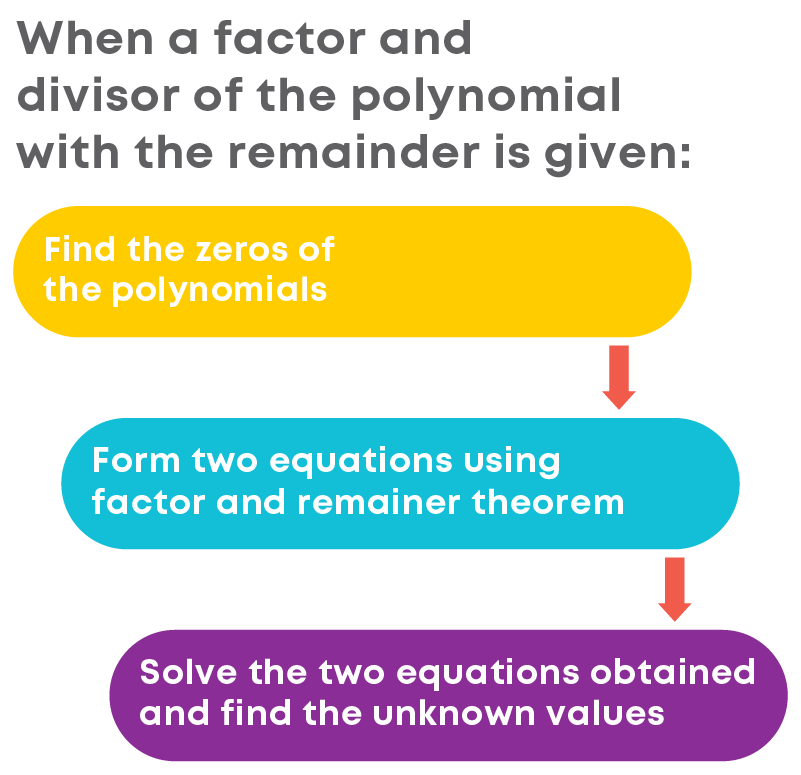#### Algebraic Identities

Let us find solutions of the equation (a + 1)2 = a2 + 2a + 1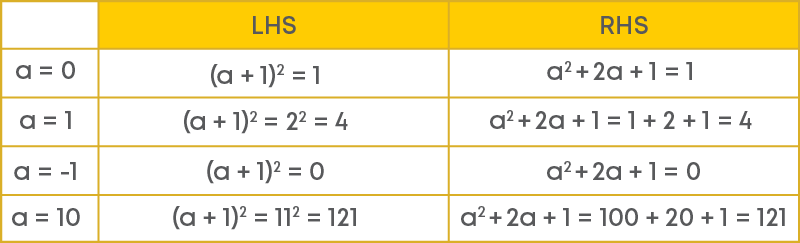‘0’, 1, -1 and 10 are solutions of the equation (a + 1)2 = a2 + 2a + 1. Now, check whether 3 is a solution of the equation. How do you check it? Substitute 3 in the place of ‘a’ on the LHS and the RHS separately and compare the values.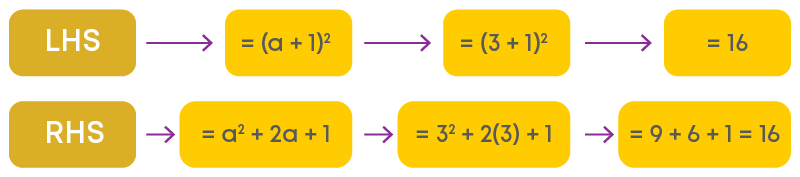So, what do you conclude? 3 also is a solution of the equation (a + 1)2 = a2 + 2a + 1.

Choose whichever number you want and check whether that number is a solution of the equation. You will find that any value of the variable is a solution of the equation. Thus, (a + 1)2 = a2 + 2a + 1 is an equality which is true for any value of the variable ‘a’. Such an equality is called an identity.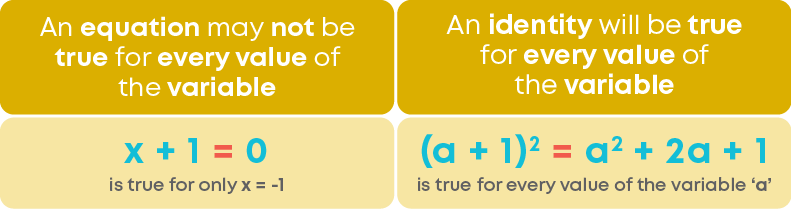Find (2p + 3)(2p – 3) using appropriate identity.

Step 1: Use the identity (a + b)(a – b) = a2 – b2

Step 2: Substitute ‘a’ by 2p and ‘b’ by 3.

(2p + 3)(2p – 3) = (2p)2 – 32

= 4p2 – 9

Recall these standard algebraic identities: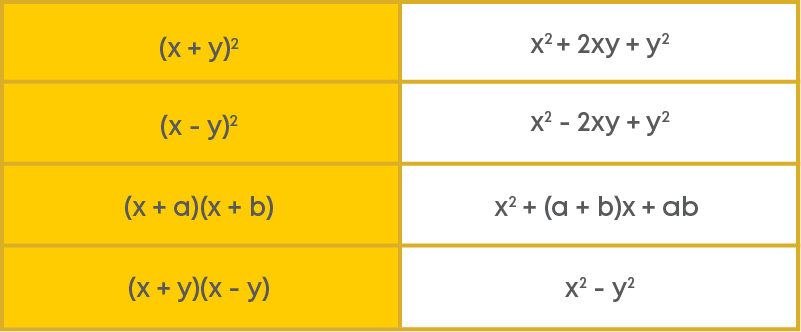Find the product of (x + 1) and (x + 2) using the identity (x + a)(x + b) = x2 + (a + b)x + ab.

Just substitute ‘a’ by ‘1’ and ‘b’ by ‘2’ in the given identity.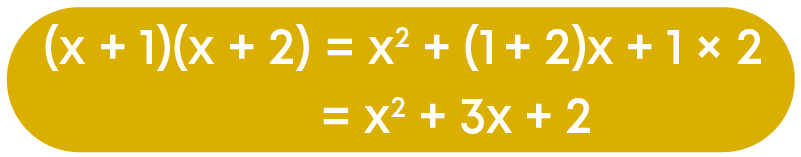Now, what do we do to find the product of (x + 1) and (x – 1)? Use the identity (a + b)(a – b) = a2 – b2Let us use identities to evaluate numbers without multiplying directly. Consider 73 × 71. It can be expressed as (70 + 3) × (70 + 1).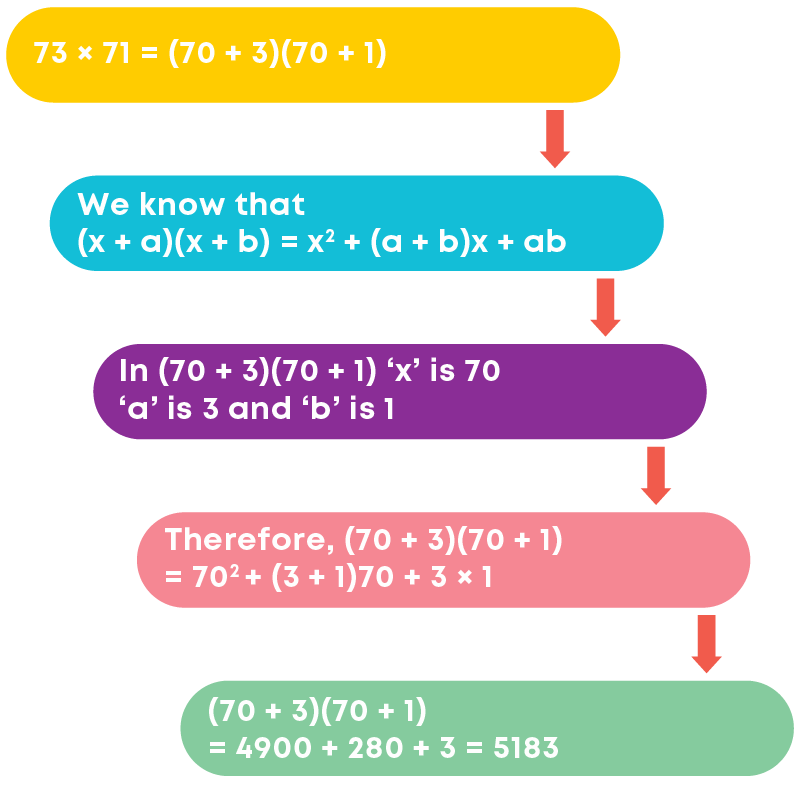Let us learn how to factorise polynomials using identities. Example: factorise 9x2 – 12x + 4.

We can rewrite this as (3x)2 – 2(3x)(2) + 22

Comparing the given expression with a2 + 2ab + b2, we get a = 3x and b = 2.

So, using the identity (a + b)2 = a2 + 2ab + b2, we get (3x)2 – 2(3x)(2) + 22 = (3x – 2)2

= (3x - 2)(3x – 2)

You know the four basic identities. Did you notice that all identities involve products of binomials? Let us now extend the Identity (I) to a trinomial x + y + z. we shall compute the square of x + y + z by using Identity I. Let us take x + y = t.Hence, we get the following identity,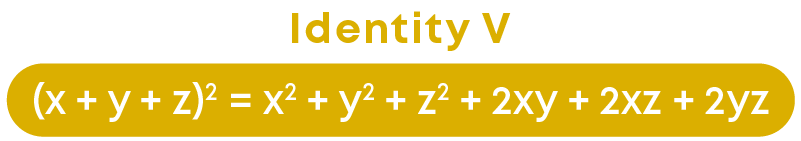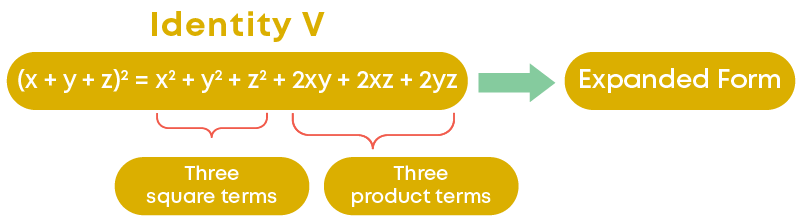In the above identity, we see that the expansion of (x + y + z)2 consists of three-square terms and three product terms.

Also, we call the right-hand side expression the expanded form of the left-hand side expression.

Factorise 9x2 + 4y2 + 16z2 + 12xy – 16yz – 24xz

First thing that we observe here is that there are two terms with negative sign, – 16yz and – 24xz. Is there anything common in both terms? Yes, the variable ‘z.’ So, let us write 16z2 as (-4z)2.

Thus, we have, 9x2 + 4y2 + 16z2 + 12xy – 16yz – 24xz

= (3x)2 + (2y)2 + (-4z)2 + {2 × (3x) × (2y)} + {2 × (2y) × (-4z)} + {2 × (3x) × (-4z)}

This is in the form of {3x + 2y + (-4z)}2

Hence, 9x2 + 4y2 + 16z2 + 12xy – 16yz – 24xz can be factorised as {3x + 2y + (-4z)}2

Or

{3x + 2y + (-4z)}{3x + 2y + (-4z)}

Now, let us understand how to find the cube of a binomial. Let us find (x + y)3.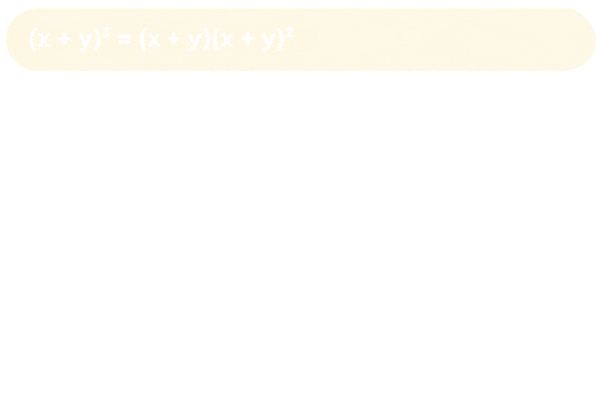We finally get,If we replace y by -y in the above identity, what do we get? We get:Let us write (3a + 5b)3.

Comparing the given expression with (x + y)3, we get x = 3a and y = 5b.

So, using Identity VI we have,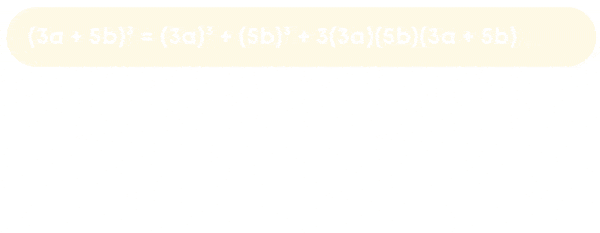We can use the same method to evaluate the cube of a number. Let us see how. Evaluate (102)3 using an identity.Now let us factorise 8x3 + y3 + 12x2y + 6xy2.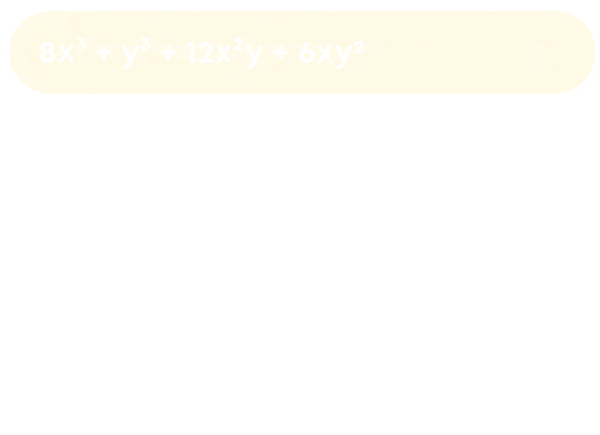Now we shall learn about identity for the sum of the cubes of three quantities minus three times their product. To obtain the identity VIII, we consider the following:

(x + y + z)(x2 + y2 + z2 – xy – yz – zx)

On expanding, we get the product as

x(x2 + y2 + z2 – xy – yz – zx) + y(x2 + y2 + z2 – xy – yz – zx) + z(x2 + y2 + z2 – xy – yz – zx)

= x3 + xy2 + xz2 – x2 y – xyz – x2 z + yx2 + y3 + yz2 – xy2 – y2 z – zxy + zx2 + zy2 + z3 – zyx – yz2 – z2 x

After simplification we get, x3 + y3 + z3 – 3xyz

So, we obtain the following identity: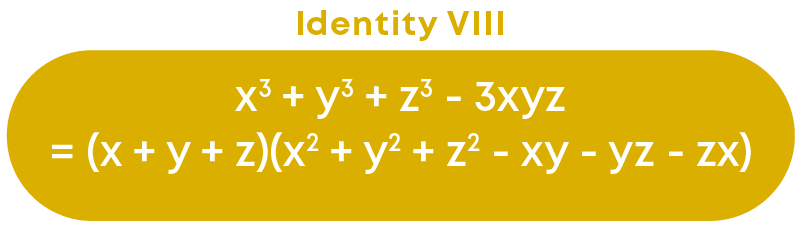Using identity VIII, we know that a3 + b3 + c3 – 3abc = (a + b + c)(a2 + b2 + c2 – ab – bc – ac)

What happens if a + b + c = 0?

a3 + b3 + c3 – 3abc = 0 × (a2 + b2 + c2 – ab – bc – ac)

a3 + b3 + c3 – 3abc = 0

a3 + b3 + c3 = 3abc

Factorise 8x3 + 27y3 + z3 – 18xyz

Step 1: Rewrite/rearrange the given terms.

8x3 + 27y3 + z3 – 18xyz can be rewritten as (2x)3 + (3y)3 + (z)3 – 3(2x)(3y)(z)

Step 2: Use identity and compare the given terms.

Upon using the identity, we get: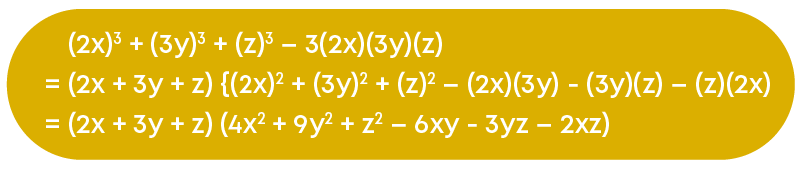#### Common Errors

The following are topics in which students make common mistakes when dealing with polynomials:

• 1. Difference between algebraic expressions and polynomials
• 2. Number of terms in a polynomial
• 3. Zero polynomial and zero of a polynomial
• 4. Remainder theorem and Factor theorem
• 5. Identities

#### Difference Between Algebraic Expressions And Polynomials

All polynomials are algebraic expressions, but all algebraic expressions are not polynomials. For example, 3x2 + x + 7 x is an algebraic expression, but not a polynomial. It is not a polynomial since it has a term (i.e., 7 x ) in which the power of the variable is a negative integer (i.e., 7 x = 7x-1).

Remember, a polynomial cannot have a variable with a negative power or fractional power, but it can have a fractional or negative or irrational coefficient or constant term.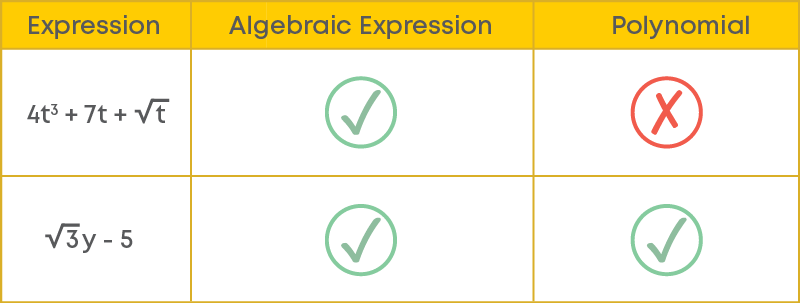#### Number Of Terms In A Polynomial

The term ‘polynomial’ is made up of two words ‘poly’ and ‘nomial’ which mean ‘many’ and ‘term’, respectively. It doesn’t mean that a polynomial should have many number of terms. A polynomial can have any number of terms, that is, one, two, three or more than three terms.#### Zero Polynomial And Zero Of A Polynomial

A zero polynomial is a polynomial consisting of only one term which is the constant 0. That is, a zero polynomial is simply the number 0. A zero of a polynomial is a value of the variable at which the value of the polynomial is 0. Remember, a zero of a polynomial need not be 0, but it can be any real number.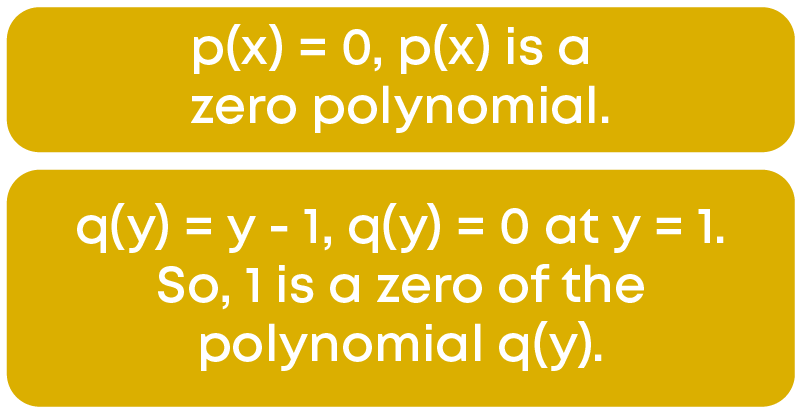#### Remainder Theorem And Factor Theorem

Remainder theorem and factor theorem are applicable only when the divisor is a linear polynomial.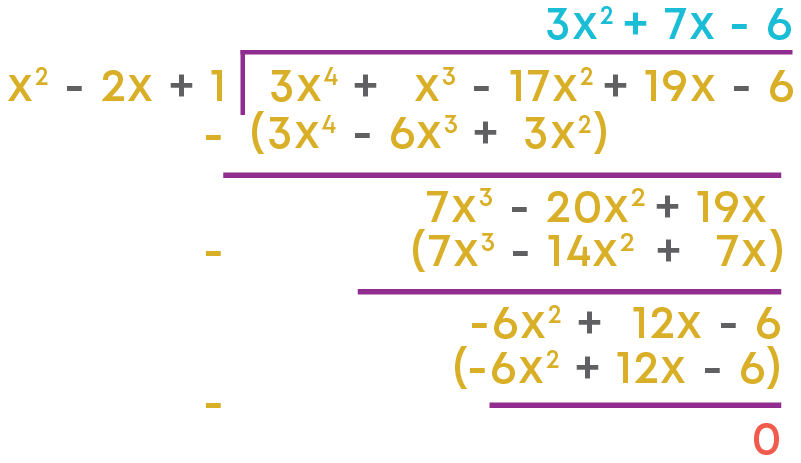When the polynomial 3x4 + x3 – 17x2 + 19x – 6 is divided by x2 – 2x + 1, the remainder is 0. This means that x2 – 2x + 1 is a factor of 3x4 + x3 – 17x2 + 19x – 6.

Here, the divisor x2 – 2x + 1 is a quadratic polynomial.

-1 is a zero of the polynomial x2 – 2x + 1.

At -1, the value of the polynomial 3x4 + x3 – 17x2 + 19x – 6 is given by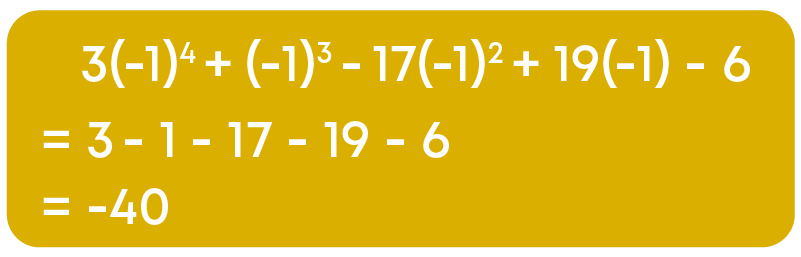That is, at a zero of the divisor, the value of the polynomial is -40, but looking at the actual division, it is evident that the remainder is 0.

That means the remainder theorem and the factor theorem are not applicable when the divisor is not a linear polynomial.

#### Identities

x3 + y3 is different from (x + y)3 and x3 – y3 is different from (x – y)3

Similarly, x2 + y2 + z2 is different from (x + y + z)2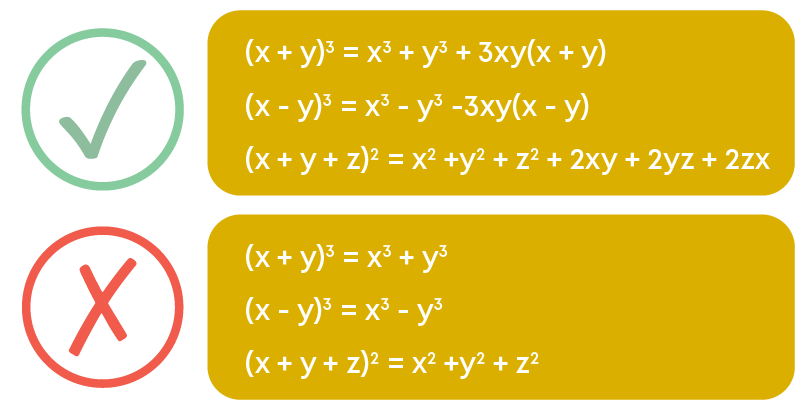#### Conclusion

Now that you learnt about polynomials, can you solve this riddle?

Given below is the layout of a house.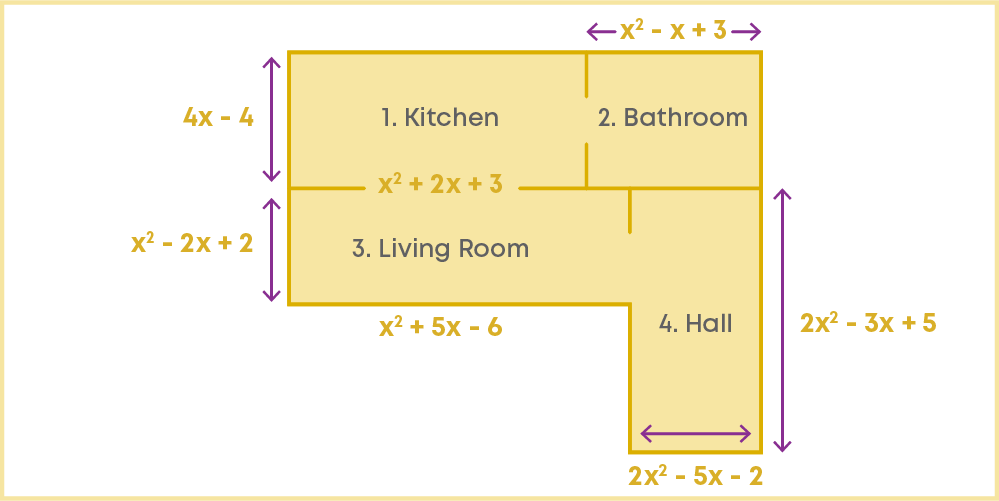The areas of each room are given but they are jumbled. Match the room with its correct area:

4x3 – 8x2 + 8x – 12 sq. units

x4 + 3x3 – 14x2 + 22x – 12 sq. units

4x3 + 4x2 + 4x – 12 sq. units

4x4 – 4x3 – 9x2 – 31x – 10 sq. units#### Arpana

Author

Arpana is an education specialist with years of teaching Math and developing content. She previously worked as a freelance content developer, developing lesson plans for a reputed publisher of text books and content for various educational companies. She is an enthusiastic teacher who has taught students from India, the UK, and New Zealand.